Next: Defining Functions Up: Getting Started With Previous: Maple Commands

## Defining and Manipulating Expressions.

In Maple you can give an expression a name so that you can refer to it by the name later on. The following example shows how to define the expression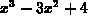and denote it by the name p.

```  > p:= x^3-3*x^2+4;
```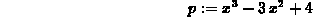Names can be longer than one letter, e.g. dist would be a valid name for an expression. In general, Maple statements must be terminated with a semicolon (;). The main exception is when you are using the on-line help, as described below. The ``:='' notation is becoming common in Mathematics and Computer Science; in English it means ``is defined to be''.

Maple understands common mathematical notation in defining expressions, as shown in Table.Table:  Binary arithmetic operators

Note that Maple doesn't allow implied multiplication. The string 3x would be interpreted as the name `3x' and not as 3 times x!

Maple has many commands for manipulating expressions. For example the factor command finds the factors involving rational roots of a polynomial and prints out the (partially) factored result. The command for substituting one expression into another has the syntax

> subs(variable = value,expression);

where variable is a variable that appears in the expression expression and value can be a number or an expression. The two examples below demonstrate subtituting the number 1 and the expressionfor x in the expression p we defined in the previous example.

```  > subs(x=1,p);
```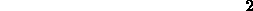```  > subs(x=t^2,p);
```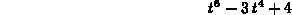would result in the expression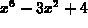. For more commands involving expressions, see the on-line help or the section on Maple commands.

William W. Farr
Mon Aug 28 09:31:56 EDT 1995